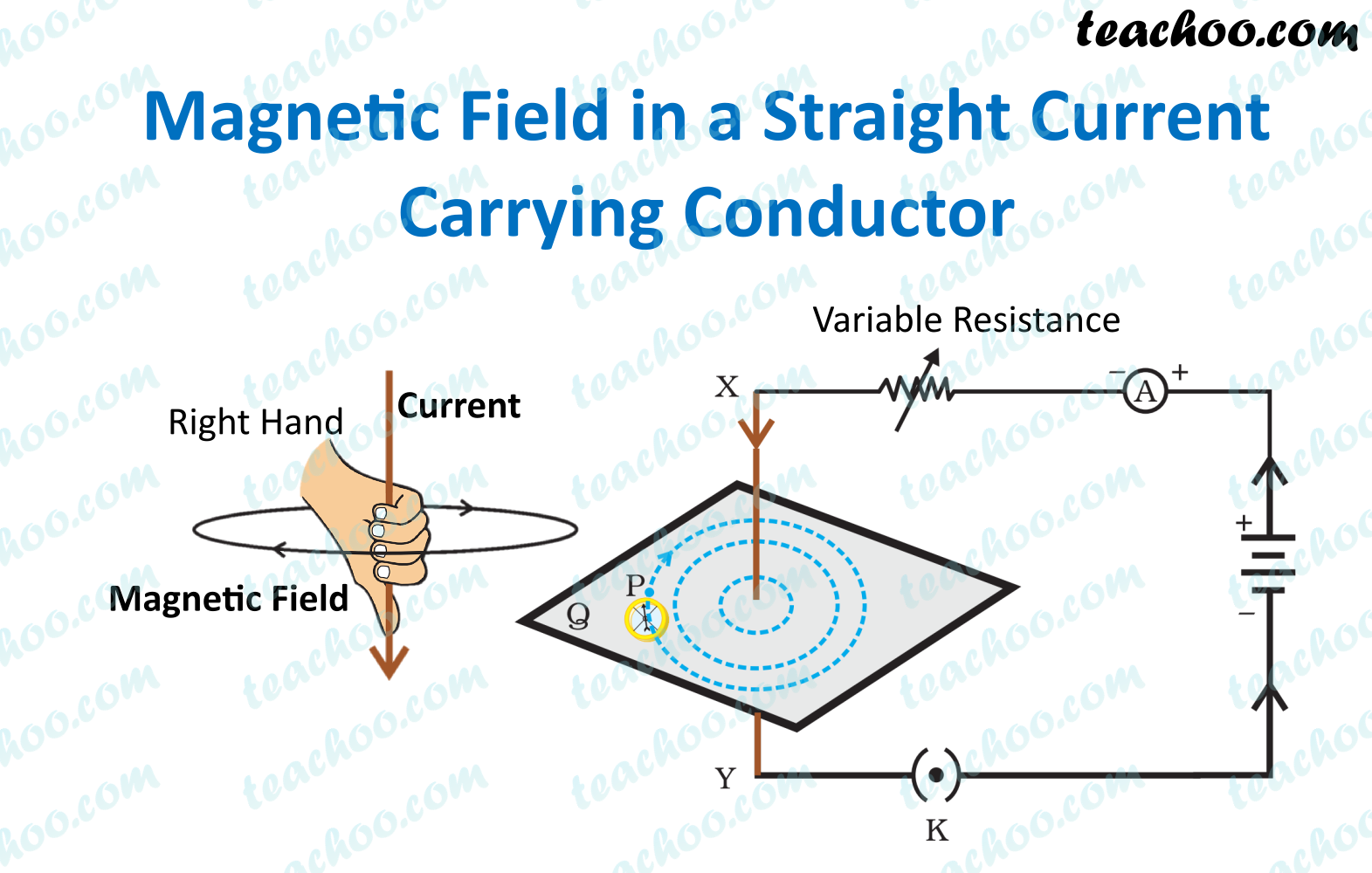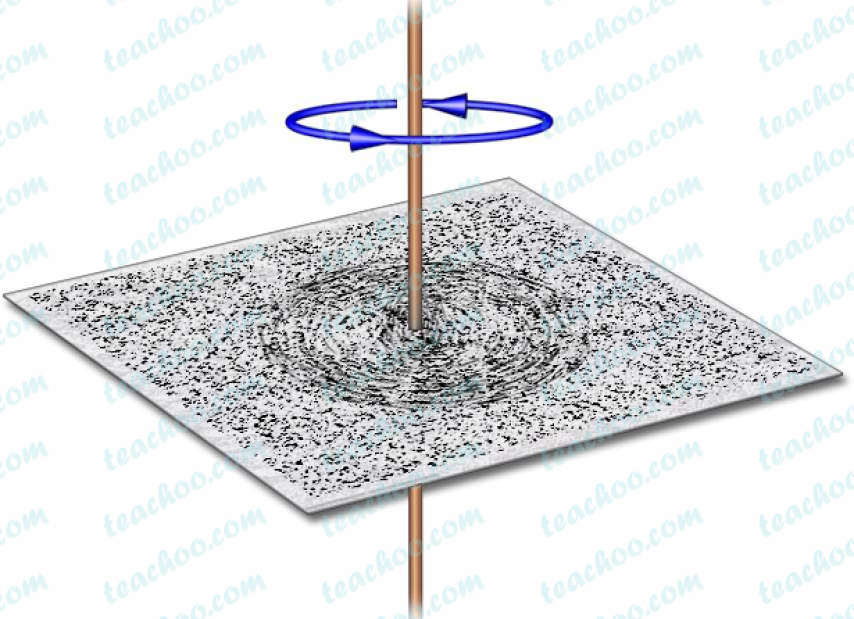Concepts

Class 10
Chapter 13 Class 10 - Magnetic Effects of Electric Current

When electric current is carried by a straight wire, it produces magnetic field lines in the form of concentric circles.## Important Points

### Magnetic field lines produced are in the form of concentric circles

Note : Circles with a common center are called concentric circles

Activity -

Suppose we make electric circuit using straight copper wire

We bring iron fillings near it and switch on the circuit

The iron fillings arrange themselves in form of concentric circles around copper wire

This shows that magnetic field lines produced by a straight conductor (wire) is in form of concentric circlesNote -
If concentric circles are closer to each other, they denote more current
If concentric circles are wide apart, they denote less current in circuit

## Direction of Magnetic Field Depends upon Flow of Electric Current

If we bring a compass near electric circuit

If current flows from North to South, North Pole of compass would deflect towards east side

If direction of current was reversed (current now flows from South to North) compass would show deflection towards west side

Direction of magnetic field is given by Right Hand Thumb Rule

## Magnitude of electric current produced is Directly Proportional to Current

Magnetic Effect is measured with help of Magnetic Compass

If less current is passed, there is less deflection in magnetic compass needle.

If current is increased, there is more deflection in magnetic compass needle. (magnitude of deflection also increases)

## Magnitude of electric current produced is Inversely Proportional to Distance of Point from Wire

If Magnetic Compass is placed near the wire, it shows more deflection (meaning more current flows through it)

If compass is moved away, the amount of deflection decreases

## Questions

NCERT Question 1 - Which of the following correctly describes the magnetic field near a long straight wire?

(a) The field consists of straight lines perpendicular to the wire.

(b) The field consists of straight lines parallel to the wire.

(c) The field consists of radial lines originating from the wire.

(d) The field consists of concentric circles centred on the wire.

Learn in your speed, with individual attention - Teachoo Maths 1-on-1 Class